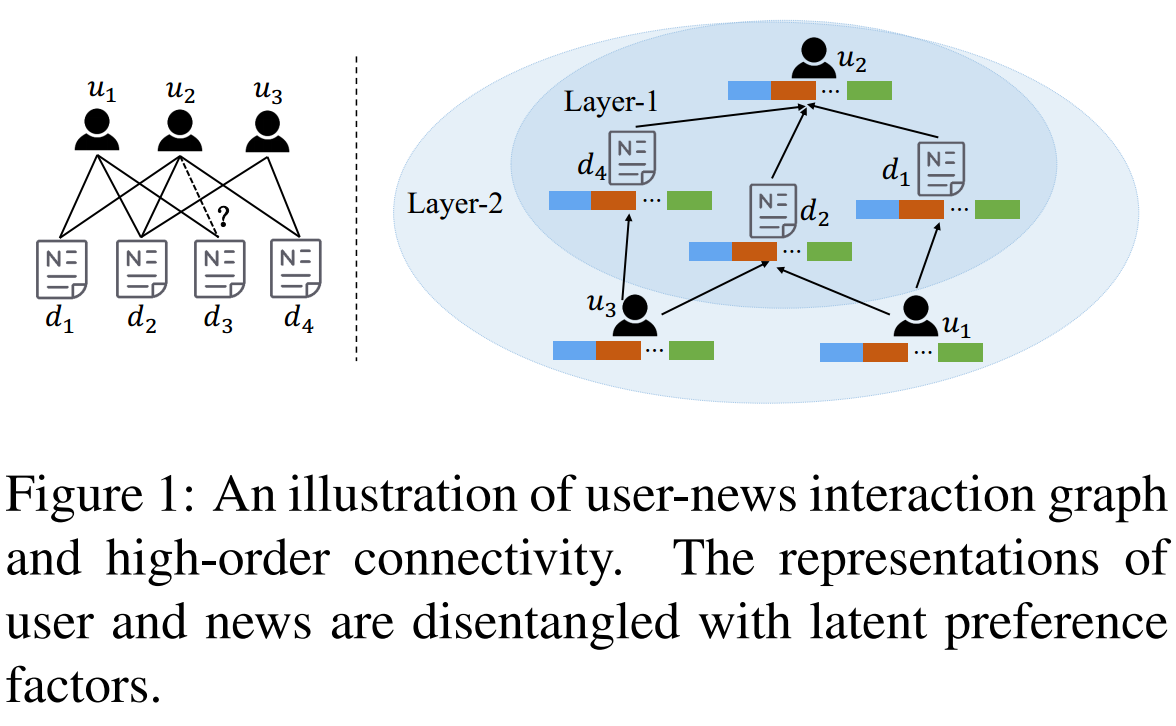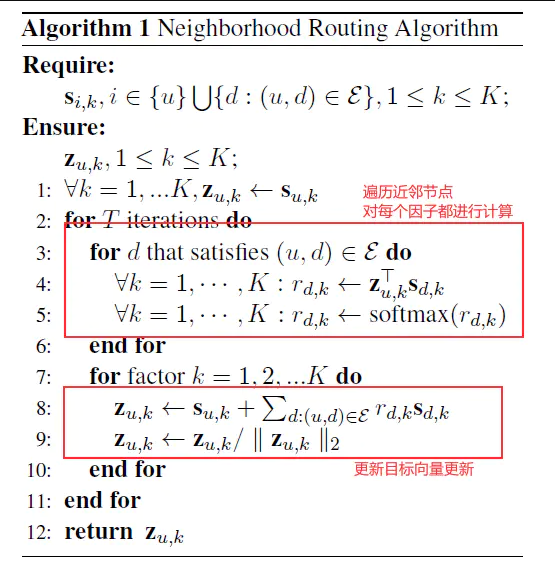Hope is a dangerous thing, but I have it.

# 【论文阅读】Graph Neural News Recommendation with Unsupervised Preference Disentanglement (ACL 2020)

News Recommendation：给定一系列成对的user-news的数据，表示user之前看过news，预测对于一个未见过的news，user是否会看。

## Motivation

• 充分利用用户的历史信息，做personalized News Recommendation
• 利用文本内容，得到user和news的好的表示
• 利用外部知识
• 利用topic信息本文方法：
• 考虑高阶的user-news之间的关系（News Recommendation中很少）。
• 考虑neighbor对当前的作用时，不应该将所有的neighbor等价看待，而应该给予不同的权重。同时，用户的倾向（preference）具有多样性，可以进一步细分在不同preference下周围neighbor的重要性。所以本文使用了disentangled representations，同时可以增强可解释性。（News Recommendation第一次）
PS：用推荐已经出现了类似的研究（何向南SIGIR2020的DGCF），跟本文很相似。

## Method

1. 构建二分图：
• 节点：
i. user：随机初始化的向量
ii. news：输入news的title和profile（包括entity和对应的type），使用PCNN分别得到表示，concat作为news的表示。
• 边：user-news之间的click关系，如果一个user点了一个news，则两者之间有一条边。
1. 图的更新$$y_u = Conv(h_u,{h_d:(u,d)\in \varepsilon })$$
• 维度变换：假定有K个factor，将表示映射到第K个factor的子空间中：
$$s_{i,k} = \frac{ReLU(W_k^Th_i+b_k)}{||ReLU(W_k^Th_i+b_k)||_2}$$
• 表示更新：当更新第k个factor相关的表示时，让与u在第k个factor上相关性高的文本贡献大 - Neighborhood routing algorithm。$$r^{(t)}_{d,k} = \frac{exp(z^{(t)T}_{u,k}s_{d,k})}{\sum_{k'=1}^{K}{exp(z^{(t)T}_{u,k}s_{d,k})}}$$
$$z^{(t+1)}_{u,k} = \frac{s_{u,k}+\sum_{d:(u,d) \in \varsigma}r^{(t)}_{d,k}s_{d,k})}{||s_{u,k}+\sum_{d:(u,d) \in \varsigma}r^{(t)}_{d,k}s_{d,k})||_2}$$
• 得到最终的表示并计算相似度得到最终的可能性输出：
$$y'_u = W^{(L+1)T}y^{(L)}_u + b^{(L+1)}$$
$$\hat{s}\langle u,d \rangle = y'^T_uy'_d$$
$$\hat{y}_{u,d} = \sigma(\hat{s} \langle u,d \rangle)$$
1. Preference Regularizer：为了使得不同的factor得到的表示不同，新增了一个预测任务，即对第k个factor得到的表示进行分类，期望分在第k类的概率最大。
2. 模型训练：
• Loss 1: News Recommendation的loss
$$L_1 = -[y_{u,d}ln(\hat{y}_{u,d}) + (1-y_{u,d})ln(1-\hat{y}_{u,d})]$$
• Loss 2: Preference Regularizer的loss
$$L_2 = -\frac{1}{K}\sum_{k=1}^{K}{\sum_{i \in {u,d}}{lnP(k|z_{i,k})[k]}}$$
• 最终的loss：
$$L = \sum_{(u,d) \in T_{train}}{((1-\lambda)L_1 + \lambda L_2) + \eta ||\Theta||}$$

1. 和SOTA对比
2. 消融实验
3. 可解释性分析
4. 参数分析